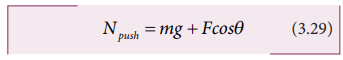Home | | Physics 11th std | To Move an Object - Push or pull? Which is easier?

# To Move an Object - Push or pull? Which is easier?

Physics : Laws of Motion : To Move an Object - Push or pull? Which is easier?

To Move an Object - Push or pull? Which is easier?

When a body is pushed at an arbitrary angle θ (0 to π/2) , the applied force F can be resolved into two components as F sinθ parallel to the surface and F cosθ perpendicular to the surface as shown in Figure 3.26. The total downward force acting on the body is mg + Fcosθ. It implies that the normal force acting on the body increases. Since there is no acceleration along the vertical direction the normal force N is equal toAs a result the maximal static friction also increases and is equal toEquation (3.30) shows that a greater force needs to be applied to push the object into motion.When an object is pulled at an angle θ, the applied force is resolved into two components as shown in Figure 3.27. The total downward force acting on the object isEquation (3.31) shows that the normal force is less than Npush. From equations (3.29) and (3.31), it is easier to pull an object than to push to make it move.

Study Material, Lecturing Notes, Assignment, Reference, Wiki description explanation, brief detail
11th Physics : UNIT 3 : Laws of Motion : To Move an Object - Push or pull? Which is easier? |